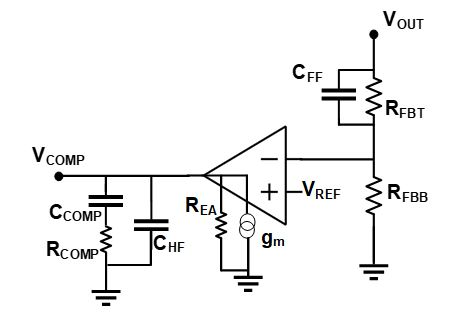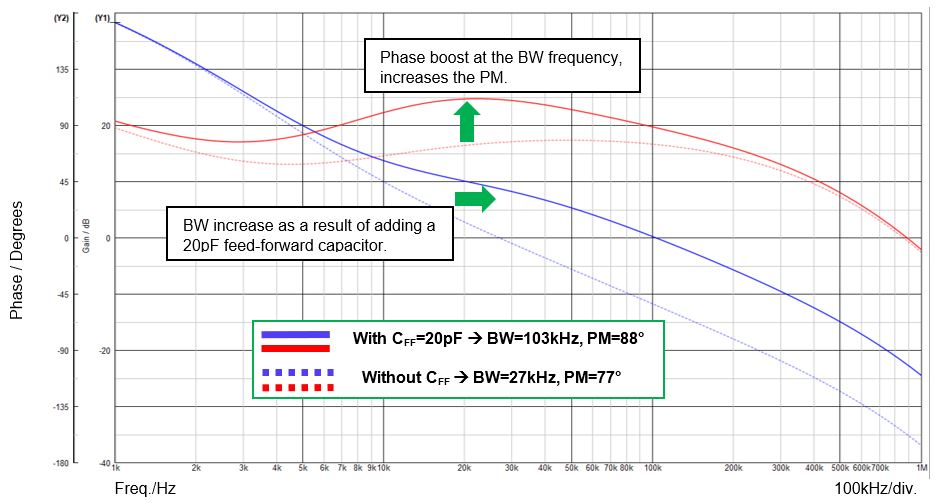# 用集成补偿网络来评估降压稳压器的瞬态性能## 峰值电流模式降压稳压器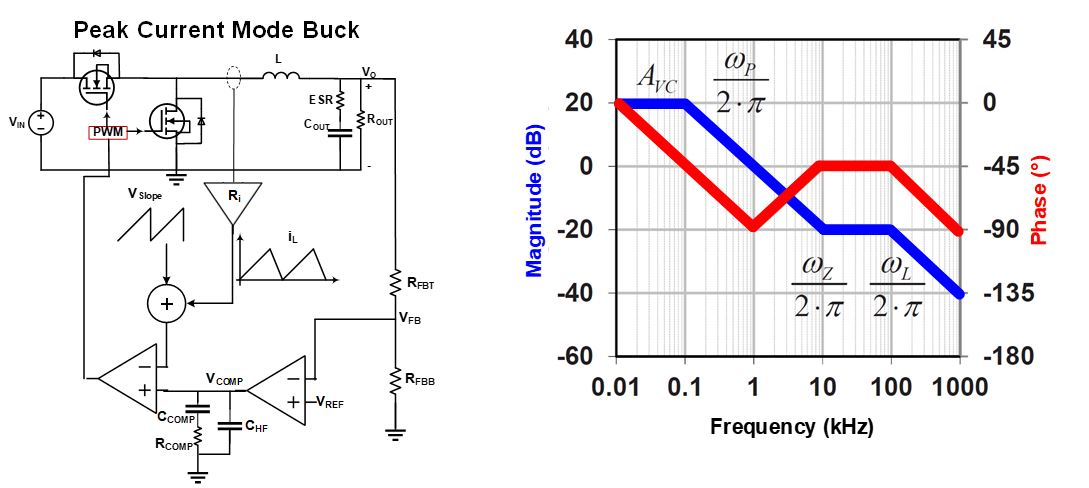$$ω_P \approx {1 \over C_{OUT} \times R_{OUT}}$$ $$ω_P \approx {K_m \times R_i \over L}$$

$$R_i=A \times R_s$$

$$K_m \approx {V_{IN} \over {V_{SLOPE}}}$$

PCM 控制降压稳压器(ωZ)中的 ESR 零点频率可以用公式 (5) 计算：

$$ω_Z \approx {1 \over C_{OUT} \times ESR}$$

## 典型II 类补偿网络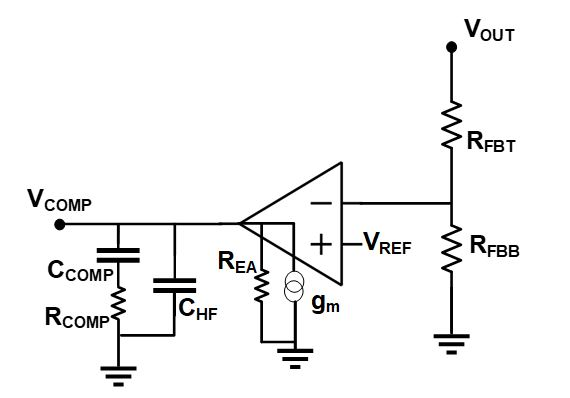COMP-Z 的频率可以用公式 (6) 计算：

$$ω_{COMP-Z} \approx {1 \over R_{COMP} \times C_{COMP}}$$

COMP-P 的频率可以用公式 (7) 计算：

$$ω_{COMP-Z} \approx {1 \over R_{COMP} \times C_{HF}}$$

## 内部补偿网络评估指南

$$BW = 0.1 \times f_{SW}$$

$$0.1 \times BW < f_{COMP-Z} < 0.2 \times BW$$

$$f_{COMP-P} = {f_{SW} \over 2}$$

$$C_{HF} < 4\% \times C_{COMP}$$

## 具有可配置开关频率的器件

1. 根据最小可配置开关频率设置COMP-Z。
2. 根据最大可配置开关频率设置COMP-P。

COMP-Z 是根据最小可配置开关频率设置的，因为随着开关频率的增加，电感器尺寸会成比例地缩小。观察PCM 降压稳压器功率级中的第二个极点（由公式2表示）可以发现，极点频率 (ω_L)随着电感 (L) 的降低而增大。随着 ωL 的增大，由该极点引起的相位延迟也被进一步推离 BW 频率。由该极点引起的负相位减少导致系统整体相位的增加，继而导致系统PM增加。

## 案例研究- MPQ4430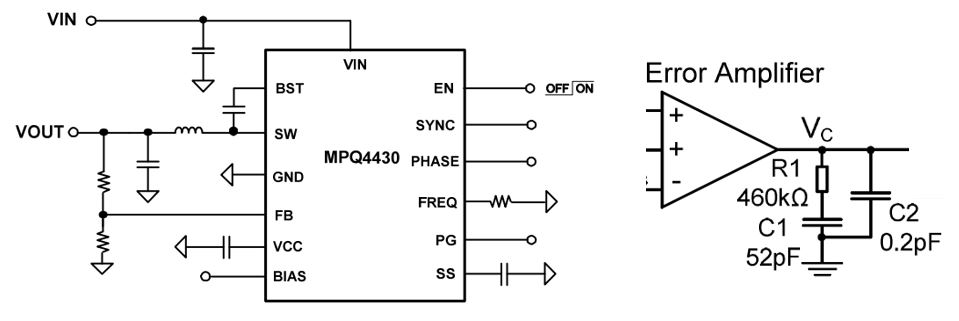## 图 4：三种开关频率下 MPQ4430 波特图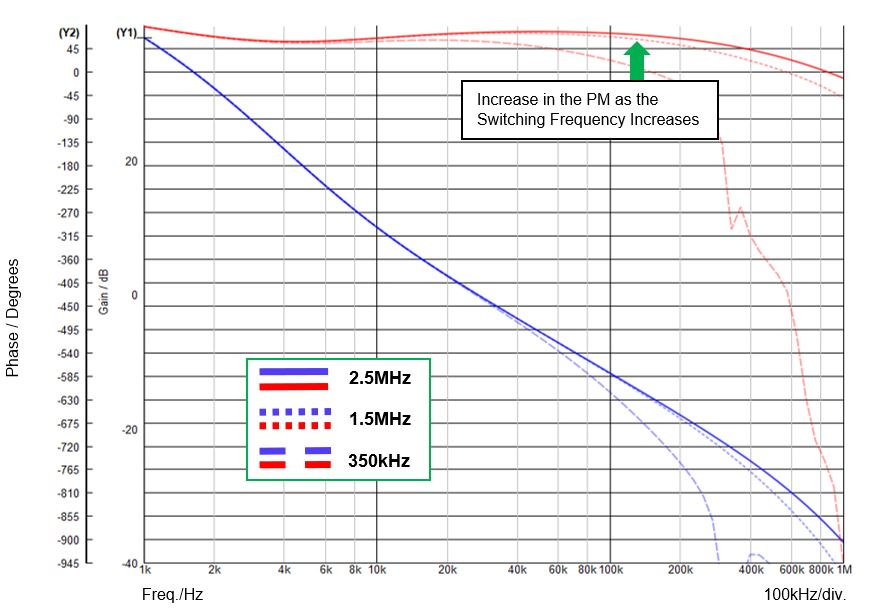开关频率 电感 带宽(BW) 相位裕度(PM) 350kHz 8µH 27kHz 60° 1.5MHz 1.8µH 27kHz 70º 2.5MHz 1µH 27kHz 77º